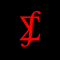Words Input Calculator
See on store
Category
Store
Last Updated
2022, Aug 24
Publisher
Visibility Score
0

Feb, 2023
Worldwide

Feb, 2023
Worldwide

## Description

Words input calculator app to perform all the calculations, ranging from basic arithmetic to complex mathematics. Getting this calculator app will give you the ability to perform arithmetic operations with word inputs on numbers. This calculator can calculate and solve many problems, from addition, subtraction, multiplication, percentages, points, division mathematical calculations and many more using one of the arithmetic methods. Compatible for all iOS devices.